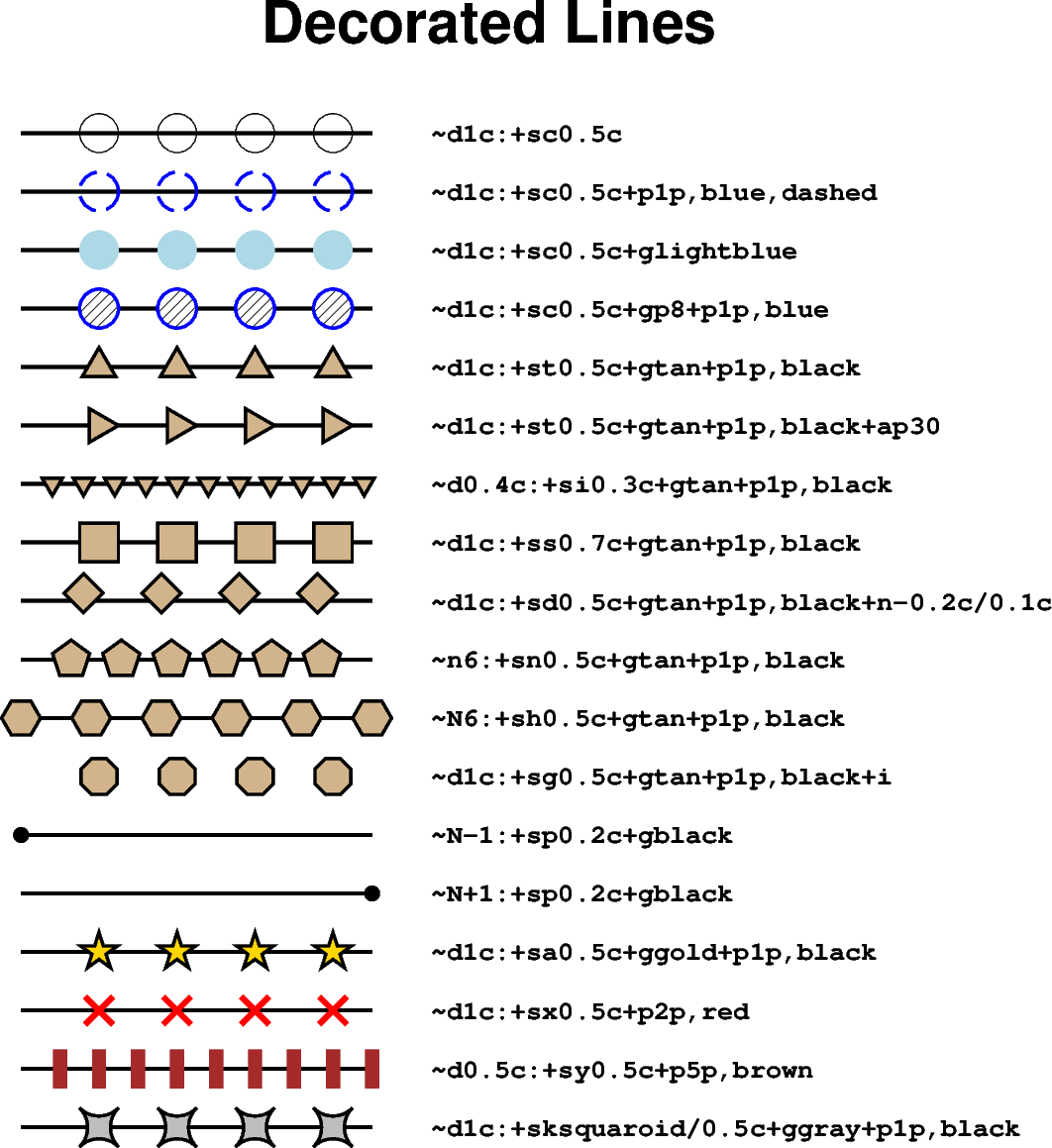# Decorated lines

To draw a so-called decorated line, i.e., symbols along a line or curve, use the `style` parameter of the `pygmt.Figure.plot` method with the argument `"~"` and the desired modifiers. A colon (`":"`) is used to separate the algorithm settings from the symbol information. This example shows how to adjust the symbols. Beside the built-in symbols also custom symbols can be used. For modifying the main decorated line via the `pen` parameter, see the Line styles example. For details on the input data see the upstream GMT documentation at https://docs.generic-mapping-tools.org/latest/plot.html#s. Furthermore, there are so-called line fronts, which are often used to plot fault lines, subduction zones, or weather fronts; for details see the Line fronts example.```import numpy as np
import pygmt

# Generate a two-point line for plotting
x = np.array([1, 4])
y = np.array([24, 24])

fig = pygmt.Figure()
fig.basemap(region=[0, 10, 0, 24], projection="X15c", frame="+tDecorated Lines")

# Plot different decorated lines
for decoline in [
# Line with circles ("c") of 0.5 centimeters radius in distance of
# 1 centimeter
"~d1c:+sc0.5c",
# Adjust thickness, color, and style of the outline via "+p"
# Here, we plot a 1-point thick, blue, dashed outline
"~d1c:+sc0.5c+p1p,blue,dashed",
# Add a fill color using "+g" with the desired color
"~d1c:+sc0.5c+glightblue",
# To use a pattern as fill append "p" and give the pattern number
"~d1c:+sc0.5c+gp8+p1p,blue",
# Line with triangles ("t")
"~d1c:+st0.5c+gtan+p1p,black",
# Line with inverse triangles with a size of 0.3 centimeters in a
# distance of 0.4 centimeters
"~d0.4c:+si0.3c+gtan+p1p,black",
# Line with squares ("s") with a size of 0.7 centimeters in a distance of
# 1 centimeter
"~d1c:+ss0.7c+gtan+p1p,black",
# Shift symbols using "+n" in x and y directions relative to the main
# decorated line
"~d1c:+sd0.5c+gtan+p1p,black+n-0.2c/0.1c",
# Give the number of equally spaced symbols by using "n" instead of "d"
"~n6:+sn0.5c+gtan+p1p,black",
# Use upper-case "N" to have symbols at the start and end of the line
"~N6:+sh0.5c+gtan+p1p,black",
# Suppress the main decorated line by appending "+i"
"~d1c:+sg0.5c+gtan+p1p,black+i",
# To only plot a symbol at the start of the line use "N-1"
"~N-1:+sp0.2c+gblack",
# To only plot a symbol at the end of the line use "N+1"
"~N+1:+sp0.2c+gblack",
# Line with stars ("a")
"~d1c:+sa0.5c+ggold+p1p,black",
# Line with crosses ("x")
"~d1c:+sx0.5c+p2p,red",
# Line with (vertical) lines or bars ("y")
"~d0.5c:+sy0.5c+p5p,brown",
# Use custom symbol ("k") "squaroid" with a size of 0.5 centimeters
"~d1c:+sksquaroid/0.5c+ggray+p1p,black",
]:
y = y - 1.2  # Move current line down
fig.plot(x=x, y=y, style=decoline, pen="1.25p,black")
fig.text(
x=x[-1],
y=y[-1],
text=decoline,
font="Courier-Bold",
justify="ML",
offset="0.75c/0c",
)

fig.show()
```

Total running time of the script: ( 0 minutes 3.802 seconds)

Gallery generated by Sphinx-Gallery